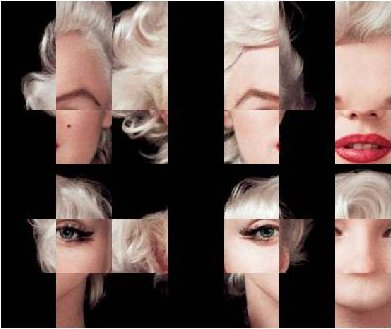# Face To Face With Marilyn Monroe

Symmetry is what we see at a glance (Blaise Pascal)

Ladies and gentlement, the beautiful Marilyn Monroe:There are several image processing packages in R. In this experiment I used `biOps`, which turns images into 3D matrices. The third dimension is a 3-array corresponding to (r, g, b) color of pixel defined by two other dimensions. Since images are defined by matrices, you can do simple operations to produce interesting images from the original one. For example, this is what happens swaping last half of columns by first one and preserving its order:It is also very simple to generate two artificial symmetrical faces matching each half with itself as a mirror. By the way, I prefer the first one: maybe two sexy moles are better than just one.

Let’s introduce a bit of randomness. This is what happens when you take an uniform sample of rows and columns (first image) or an uniform sample of pixels of the image (second one):

And this is what happens when you divide image into blocks and mix them randomly:There is a funny and useful function called `jitter `which adds a small amount of noise to a numeric vector. What happens when you jitter every pixel of the image? As you can see, It becomes very vintage:What if you transpose matrix? What if you change every color by another one? What if you change only a small range of them? What if you sum two images? What if you translate rgb colors into a grey scale? What if …? I answered some of these questions already and results are nice as well. After all, Marilyn can be represented as a simple matrix. Or maybe not.

```library("biOps")
library("abind")
#############################################################
#############################################################
plot(x)
#############################################################
#1. Swap
#############################################################
plot(imagedata(abind(x[,(ncol(x)/2):ncol(x),], x[,1:(ncol(x)/2),] , along=2)))
dev.copy(jpeg,filename="IMG01-Swap.jpg");
dev.off ();
#############################################################
#2. Artificial Symmetrical faces
#############################################################
plot(imagedata(abind(x[,1:(ncol(x)/2),], x[,(ncol(x)/2):1,] , along=2)))
dev.copy(jpeg,filename="IMG02-Symmetric1.jpg");
dev.off ();
plot(imagedata(abind(x[,ncol(x):(ncol(x)/2),], x[,(ncol(x)/2):ncol(x),] , along=2)))
dev.copy(jpeg,filename="IMG03-Symmetric2.jpg");
dev.off ();
#############################################################
#3. Uniform sampling over axis points
#############################################################
x2   <- aperm(array(255, dim = c(3, ncol(x), nrow(x))))
rows <- sample(1:nrow(x), round(nrow(x)*0.80), replace = FALSE)
cols <- sample(1:ncol(x), round(ncol(x)*0.80), replace = FALSE)
for (i in 1:length(rows))
{
for (j in 1: length(cols))
{
x2[rows[i], cols[j],1]<-x[rows[i], cols[j],1]
x2[rows[i], cols[j],2]<-x[rows[i], cols[j],2]
x2[rows[i], cols[j],3]<-x[rows[i], cols[j],3]
}
}
plot(imagedata(x2))
dev.copy(jpeg,filename="IMG04-Uniform1.jpg");
dev.off ();
#############################################################
#4. Uniform sampling over pixels
#############################################################
m2 <- matrix(rbinom(nrow(x)*ncol(x),1,0.5),nrow(x),ncol(x))
x4<- do.call(abind, c(list(x[,,1]*m2+(m2==0)*255,x[,,2]*m2+(m2==0)*255,x[,,3]*m2+(m2==0)*255), along = 3))
plot(imagedata(x4))
dev.copy(jpeg,filename="IMG05-Uniform2.jpg");
dev.off ();
#############################################################
#6. Jittering
#############################################################
x1<-mapply(as.matrix(x[,,1]), FUN=function(x)
{z<-round(x+jitter(0, amount=50))
if(z<0|z>255) x else z})
x1 <- matrix(x1, nrow = nrow(x),ncol = ncol(x))
x2<-mapply(as.matrix(x[,,2]), FUN=function(x)
{z<-round(x+jitter(0, amount=50))
if(z<0|z>255) x else z})
x2 <- matrix(x2, nrow = nrow(x),ncol = ncol(x))
x3<-mapply(as.matrix(x[,,3]), FUN=function(x)
{z<-round(x+jitter(0, amount=50))
if(z<0|z>255) x else z})
x3 <- matrix(x3, nrow = nrow(x),ncol = ncol(x))
x4<- do.call(abind, c(list(x1,x2,x3), along = 3))
plot(imagedata(x4))
dev.copy(jpeg,filename="IMG06-Jitter.jpg");
dev.off ();
#############################################################
#7. Mosaic
#############################################################
sptr <- 6 #Row splits
rnkr <- sample(1:sptr, size = sptr, replace = FALSE)
wthr <- floor(nrow(x)/sptr) #Splits width (row)
rnkr <- as.vector(sapply(rnkr, function (x) rep(x,wthr)))
rnkr <- rnkr*10E6+seq(1, length(rnkr), by=1)
rnkr <- rank(rnkr)
sptc <- round(ncol(x)/wthr)
rnkc <- sample(1:sptc, size = sptc, replace = FALSE)
wthc <- floor(ncol(x)/sptc) #Splits width (row)
rnkc <- as.vector(sapply(rnkc, function (x) rep(x,wthc)))
rnkc <- rnkc*10E6+seq(1, length(rnkc), by=1)
rnkc <- rank(rnkc)
x2<-x[1:length(rnkr),1:length(rnkc),]
x2<-x[rank(rnkr),rank(rnkc),]
plot(imagedata(x2))
dev.copy(jpeg,filename="IMG07-Mosaic.jpg");
dev.off ();
```

## 5 thoughts on “Face To Face With Marilyn Monroe”

1.Marko Djurakic says:

Nice issue :),
Can you specify how did you define (and find) symmetry axis? Actually, I noticed that you did something with ncol(x)/2, but can you explain it in more details?

1.aschinchon says:

I developed a genetic algorithm to find the optimal symmetry axis. I recognize I find it better “by hand” than using the genetic. I am not keen on genetic algorithms because they are so sensitive to initial settings but I didn’t give up this possibility so maybe in the future I will publish a good implementation to find optimal symmetry axis automatically. I will do my besto to do it. Meanwhile I do it by hand. Thanks for your comment!

2.Michael says:

I think it’s not such good practice to include “rm(list=ls())” in a public script, especially without drawing attention to the fact that it will delete any objects from the R session.

1.aschinchon says:

Ok, you have reason. I deleted it. Thanks for yor comment.